# Oligopoly and Collusion-Micro Economics Practical Solution Sample

QUESTION

Problem 1: Oligopoly and Collusion
In the Lower Saxon town of Celle, two neighbors, Hinz and Kunz, run two breweries that
compete in quantities and serve the local market. Their cost functions are C(q1) = q1
and C(q2) = q2, where q1 denotes the amount of beer (in thousands of liters) produced by
Brewery Hinz and q2, that by Brewery Kunz. The demand for beer in the local market of
Celle is given by the inverse demand function P(Q) = 13 − Q, where prices are given in
Euros per liter and Q = q1 + q2 denotes the total quantity (in thousands of liters) traded
in the local market.

(a) Suppose that the neighbors make simultaneous decisions about quantities to be produced. Based on the inverse demand function, what are the marginal
revenues of Hinz and Kunz? What are their profit-maximizing conditions? Determine both firms’ reaction functions.

(b) For the simultaneous quantity competition considered in (a), calculate
the output levels qC1, qC2of both firms, the total market output QC and the market price P C. Calculate the firms’ profits, the total profit in this industry and the consumer surplus. Explain why this outcome is a Nash equilibrium in the game played between these two firms

(c) Now assume that both firms agree to follow a joint strategy and produce
quantities that maximize their joint profits. What will be the production levels qM1,qM2 of each firm if they colluded and equally divide the total output? What will be the market price PM? Calculate the firms’ profits, the total profit of the colluding firms and the consumer surplus.

(d) For the case of both firms’ collusive behavior considered in (c), show
what is the best response of one firm to the other firm’s decision to follow the cartel
agreement. Now assume for simplicity that Hinz and Kunz have just two possible
strategies each: either to produce the collusive output level calculated in (c) or to
maximize the own profit given what the other firm does, i.e. to follow the own
best-response function. What are the firms’ profits if one firm produces the cartel
output and the other one maximizes its own profit? Construct the payoff matrix of
this (2×2) game. Find the Nash equilibrium of this normal-form game. Does this
Nash equilibrium lead to joint profit maximization?

(e) Now assume that the market structure changes and the neighbors make
sequential decisions about their output levels. Hinz is now the Stackelberg leader
with the 1st-move advantage, and Kunz becomes the Stackelberg follower. What
is the followers’ reaction function? Calculate the profit-maximizing output level of
both firms, the market output and the market price. Explain why this outcome is a
subgame-perfect equilibrium of this game with sequential decisions. What are the
consumer surplus and the producer surplus in this Stackelberg competition game?

(f)  Summarize all your findings for all three considered market structures
in a table with market output levels, market prices, consumer surplus, producer
surplus and social welfare for each market structure. Draw a graph where you
indicate each of these three outcomes as a combination of output and price along
the market demand curve

Problem 2: General Equilibrium in an Exchange Economy
Adam’s preferences for opera tickets (good 1) and ballet tickets (good 2) can be represented by the utility function
uA(xA1, xA2) = xA1 xA2.Eve’s utility function is given byuE(xE 1, xE2) = xE1 + xE2. Here, the subscripts denote goods whereas the superscripts denote the individuals. Adam’s initial endowment consists of 6 opera tickets and 2 ballet tickets per year whereas Eve’s initial endowment is given by 4 opera tickets and 3 ballet ticket per year. Assume that Adam and Eve are the only potential traders in this

exchange economy.
(a)  Draw an Edgeworth box for this pure exchange economy with two consumers. Indicate the point of initial endowments and sketch several indifference
curves for both consumers. Which allocations are attainable in this economy? Indicate graphically the set of mutually beneficial exchanges (’lens of exchange’)

(b) Is the initial endowment Pareto-efficient? Determine the set of all
Pareto-efficient allocations and draw the contract curve. (Hint: in this economy,
some Pareto-efficient allocations may be located on the boundary of the Edgeworth
box. I.e. it may be efficient for one of the consumers to consume positive amounts
of both goods and, for the other one, only one of two goods.

demand for opera tickets and ballet tickets as functions of prices p1, p2. Is there a
price ratio such that Adam demands a positive quantity of only one of both goods?
Now consider Eve’s preferences and endowments. At which price ratio p1/p2 would
Eve demand positive quantities of both goods?

(d)  Using your results from (c), find the equilibrium price ratio between
opera tickets and ballet tickets. Clearly explain how you achieve your result

(e) Find the equilibrium allocations. Is this market equilibrium Paretoefficient? Why or why not? Illustrate your answer graphical

Problem 3: General Equilibrium and International Trade (24 points)
Assume that two countries, A and B, can produce two types of goods, white wine (good 1)
and red wine (good 2), and trade them in the international wine market. In the production
of wine, each country fully specializes as follows. Country A produces 10 million liters of
white wine while country B produces 10 million liters of red wine. Therefore, the initial
endowments (in million of liters per year) are (ωA1, ωA2) = (10, 0) and (ωB1, ωB2) = (0, 10).
In country A, all the consumers treat both types of wine as perfect substitutes. Therefore,the utility function of country A’s representative consumer is uA(xA1, xA2) = xA1 + xA2. In country B, all the consumers treat both goods as perfect complements and, therefore, the utility function of a representative consumer in country B is uB(xB1, xB= min{x1, xB2 }.

(a) Draw an Edgeworth box that represents the situation described above before trade. State the analytical condition for a Pareto-efficient allocation in such a market. Is it possible to evaluate this condition using marginal rates of substitution for both countries? What is the graphical interpretation of this Pareto-efficiency condition? Using a graphical explanation, find the set of all Pareto-efficient allocations. Indicate the set of all mutually beneficial exchanges (’lens of exchange’).

(b) Is it possible that, in a market equilibrium, country A consumes 6 million
liters of white wine and 4 million liters of red wine whereas country B consumes
4 million liters if white wine and 6 million liters of red wine? If it is a possible
equilibrium, determine the equilibrium price ratio such that the markets are cleared
in this case and draw the corresponding price line. If it cannot be an equilibrium,
why not? (Hint: consider preference heterogeneity between both countries that
results in different demands for both goods and use a graphical solution.

(c) Is it possible that, in a market equilibrium, both countries consume 5
million liters of white wine and 5 million liters of red wine? If yes, determine the
equilibrium price ratio such that the markets are cleared in this case and draw the
corresponding price line. If not, why not? (Hint: consider preference heterogeneity
between both countries that results in different demands for both goods and use a
graphical solution.

(d) Is it possible that, in a market equilibrium, country A consumes 9 million
liters of white wine and 9 million liters of red wine whereas country B consumes
the remaining 1 million liter of white wine and 1 million liter of red wine? If yes,
determine the equilibrium price ratio such that the markets are cleared in this case
and draw the corresponding price line. If not, why not? (Hint: consider preference
heterogeneity between both countries that results in different demands for both
goods and use a graphical solution.

# Problem 1

## (a)

The price for both Hinz and Kunz will be the same. Therefore, the total revenue of each will be the quantity they produce times market price.

Total revenue for Hinz = (13 – Q) * q1 = q1*(13-Q)

Total revenue for Kunz = (13 – Q) * q2 = q2*(13-Q)

Where Q = q1 + q2

Therefore, TR for Hinz = q1*(13 – q1 – q2) = -q12 + 13 q1 – q1q2

Therefore, TR for Kunz = q2*(13 – q1 – q2) = -q22 + 13 q2 – q1q2

The marginal revenue is differential of total revenue with quantity produced.

Marginal revenue for Hinz = dTR/dq1 = –2q1 + 13 – q2

Marginal revenue for Kunz = dTR/dq2 = –2q2 + 13 – q1

The profit maximising condition is when the marginal revenue for Hinz and Kunz is equal to the marginal cost. The marginal cost is 1 (differential of the total cost to quantity). Therefore, when the sum of 2q1 + q2 and 2q2 + q1 is 12 That is when both the companies make maximum profit.

The reaction function is found by equating marginal revenue to marginal cost and finding the quantity.

For Hinz,

-2q1 + 13 – q2 = 1

q1 = (12-q2)/2

For Kunz,

-2q2 + 13 – q1 = 1

q2 = (12-q1)/2

## (b)

Solving the reaction functions for Hinz and Kunz simultaneously to calculate q1 and q2:

q1c = q2c = 4 (thousand litres)

Qc = q1c + q2c = 4 + 4 = 8 (thousand litres)

Pc = 13 – Qc = 13 – 8 = 5 Euros

Firms’ profit = Hinz’ Profit = Kunz’ Profit = 5*4 – 4 = 16 (thousand Euros)

Total Profit = 16 + 16 = 32 (thousand Euros)

The consumer surplus is the area below the demand curve and above the price paid by consumers.

Consumer Surplus = 0.5*(13-5)*8 = 32

The outcome is a Nash equilibrium as it is the simultaneous solution to the best response equations for both the firms. None of the two firms have an incentive to produce any other amounts than the ones calculated as that will decrease their profits. This is the best profit that the companies can achieve. That is not the maximum profit possible though.

## (c)

After collusion both work as a single entity producing quantity QM

The total revenue = Q*(13-Q)

Marginal Revenue = 13 – 2Q

Marginal Cost = 1

Best Response: 13 – 2Q = 1

QM = 6 (thousand litres)

Therefore q1M = q2M = 3 (thousand litres)

PM = 13 – 6 = 7 euro

Firm Profit = 7*3 – 3= 18 thousand euros

Industry Profit = 6*7 – 6 = 36 thousand euros

The consumer surplus is the area below the demand curve and above the price paid by consumers.

Consumer surplus = 0.5*(13-7)*6 = 18

## (d)

Assuming one firm produces 3 thousand litres the following will be the other firm’s response:

Total revenue = q*(13-q-3) = q*(10-q)

Marginal revenue = 10-2q

Marginal cost = 1

Best Response: 10-2q = 1

q = 4.5

Market Price = 13 – 3 – 4.5 = 5.5 euros

Profit = 5.5*4.5 – 4.5= 20.25 thousand euros

Profit for firm following cartel agreement = 3*5.5 – 3 = 13.5 thousand euros

Payoff Matrix

 Hinz Cartel Output Profit Maximisation Kunz Cartel Output (18, 18) (13.5, 20.25) Profit Maximisation (20.25, 13.5) (16, 16)

The nash equilibrium of the given normal form is achieved when both follow profit maximisation strategies. That does not lead to a joint profit maximisation as in a cartel the industry profit is 36 thousand euros whereas in case of profit maximisation the industry profit is 32 thousand euros.

## (e)

Kunz is the Stakelberg follower thus it will set quantities based on the quantity q1H set by Hinz.

Revenue for Kunz = q2*(13-q1H – q2)

Marginal Revenue = -2q2 + 13 – q1H

Marginal cost = 1

Best Response Function: -2q2 + 13 – q1H = 1

q2 = (12-q1H) / 2

Hinz knows that Kunz will set quantity based on its production levels

Total revenue = q1*(13 – q1 – q2)

Where, q2 = (12-q1) / 2

TR = q1*(13 – q1 – 6 + 0.5q1) = q1*(7 – 0.5q1)

MR = 7 – q1

Best Response: 7 – q1 = 1

q1 = 6 (thousand litres)

q2 = (12-6)/2 = 3 (thousand litres)

Market Output = q1 + q2 = 6+3 = 9

Market Price = 13-9 = 4

This is a subgame perfect equilibrium as in all the subgames of the case this will be the eventual equilibrium as long as there are finite steps. Kunz will always choose to produce 3 thousand litres as long as Hinz gets the first move. It will not make sense for Hinz to produce the duopoly profit maximisation output as that is suboptimal for Hinz.

Consumer Surplus = 0.5*(13-4)*9 = 40.5

Producer Surplus = 0.5*4*9 = 18

## (f)

 Market Output Market Price Consumer Surplus Producer Surplus Social Welfare Simultaneous Decision 8 5 32 20 52 Cartel Model 6 7 18 21 39 Stackelberg Model 9 4 40.5 18 58.5

# Problem 2

## (a)

Total x1 = 6 + 4 = 10

Total x2 = 2 + 3 = 5

W is the point of initial endowment where,

x1A = 6, x2A = 2

x1B = 4, x2B = 3

For Adam, initial utility = 6*2 = 12

For Eve, initial utility = 4 + 3 = 7

UA1 = x1Ax2A = 15

UA2 = x1Ax2A = 12

UA3 = x1Ax2A = 10

UE1 = x1Ex2E = 8

UE2 = x1Ex2E = 7

UE3 = x1Ex2E = 6

P2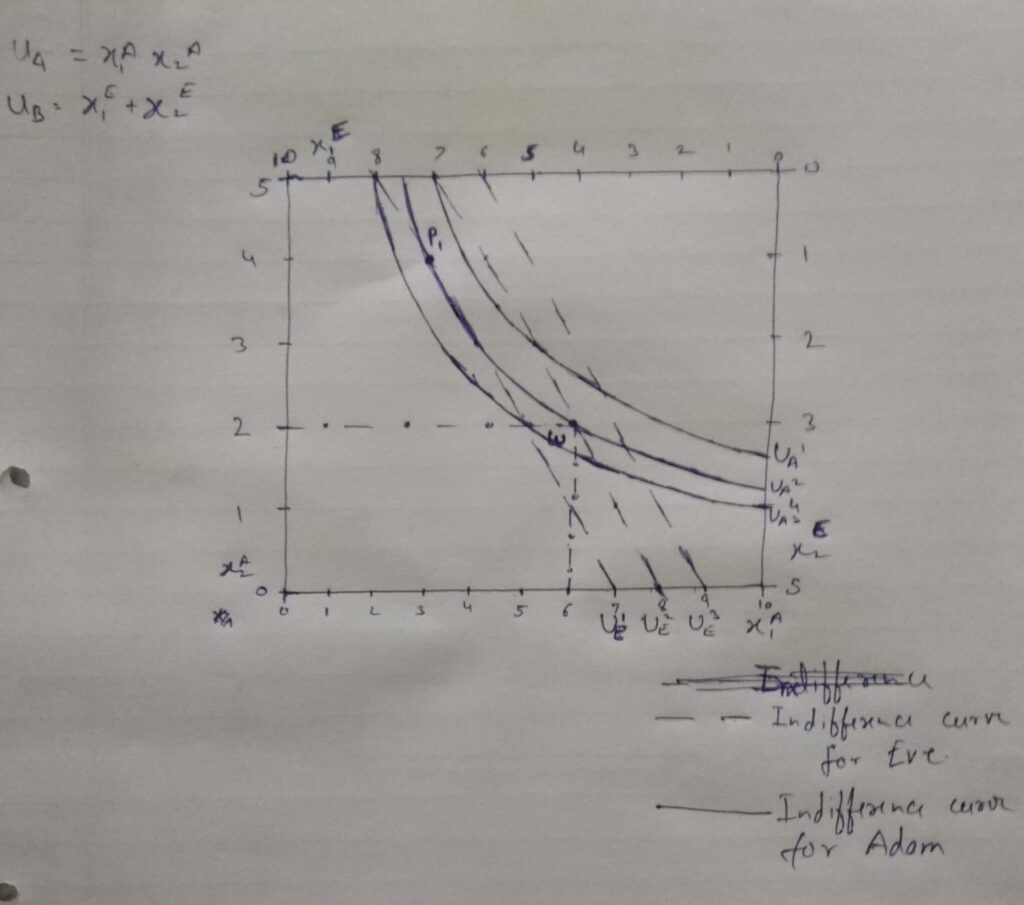All the allocations where the utility functions intersect are attainable in the economy. For example, in the diagram above point P1 is attainable in addition to w keeping the utility of Adam constant and increasing the utility of Eve. Ideally all the allocations in the Edgeworth graph are possible if there is no restriction of the utility functions.

There are two mutually beneficial exchange in the graph, P1 and P2. At P1(x1A = 3, x2A = 4, x1B = 7, x2B = 1) the utility of Adam remains constant whereas the utility of Eve increases. At P2 (x1A = 5, x2A = 3, x1B = 5, x2B = 2) the Utility of Eve remains constant whereas the utility of Adam increases. As there goods are tickets we cannot have exchanges in fractions which would have resulted in the increase in utility of both.

## (b)

The initial endowment is not pareto efficient as we saw in the previous answer that P1 and P2 are two points where either Adam or Eve can be better off without making the other worse off.

Determining Pareto efficient allocations for our economy:

MRSi1,2 = UM1i/UM2i = x2i/x1i

UM1A = dUA/dx1A = x2A

UM2A = dUA/dx2A = x1A

UM1E = dUE/dx1E = 1E

UM2E = dUE/dx2E = 1E

Equating MRSA = MRSE

x2A/ x1A = 1E/ 1E

Imposing resource Distribution

x2A/ x1A = (5 – 1)/ (10 – 1)

9x2A = 4x1A

The equation above I the equation of the contract curve and is drawn below. There are only two possible points in real scenario which can be executed: (0,0) and (9,4) from the perspective of Adam.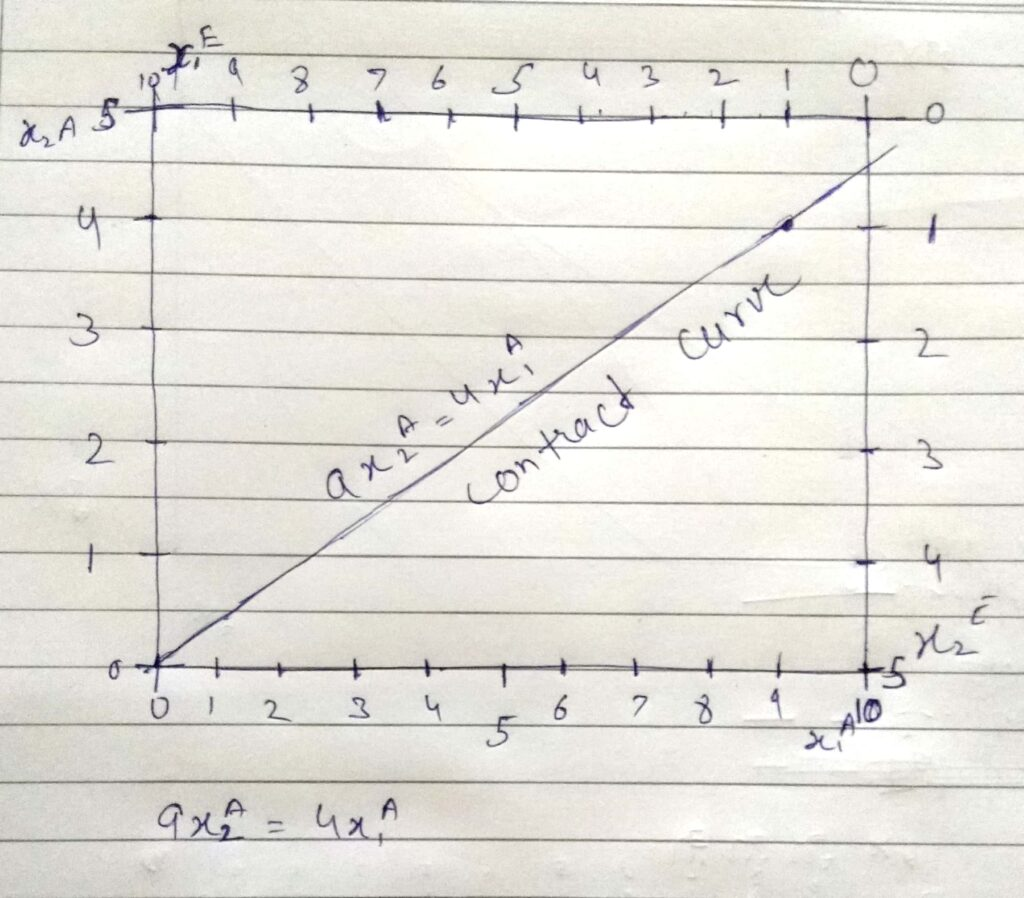## (c)

UA = x1Ax2A

p1(x1 – 6) + p2(x2 – 2) <=0

Therefore,

p1x1 + p2x2 = 6p1 + 2p2 -1

MRSA = x2/x1 = p1/p2

p1x1 = p2x2

Substituting in EQN 1

p1x1 + p1x1 = 6p1 + 2p2

x1 = 3 + p2/p1

Similarly,

p2x2 + p2x2 = 6p1 + 2p2

x2 = 1 + p1/p2

There is no such price ratio for which Adam has a positive quantity for only one of the goods.

For Eve

UA = x1E + x2E

p1(x1 – 4) + p2(x2 – 3) <=0

Therefore,

p1x1 + p2x2 = 4p1 + 3p2 -2

MRSA = 1/1 = p1/p2

p1 = p2

Substituting in EQN 2

p1x1 + p1x2 = 6p1 + 2p1

x1 = 8 – x2

For a ratio of 1 Eve will have positive demands for both the goods.

## (d)

The equilibrium price is when the demand for both the goods will be stabilised to fixed values. As the utility function by Eve is ignorant to the prices only the utility function of Adam will be used to calculate the equilibrium price ratios.

x1 = 3 + p2/­­p1 x1 <=10

x2 = 1 + p1/p2 x2 <=5

let Price ratio = k

x1 = 3 + 1/k

x2 = 1 + k

Utility functions = x1x2 = (3 + 1/k)( 1 + k) = 3 + 3k + 1/k + 1 = 4 + 3k + 1/k

Differentiating and equating to zero.

3 – 1/k2 = 0

k = SQRT(1/3)

Therefore p1/p2 = SQRT(1/3)

## (e)

The Point P1 andP2 in the graph below are the equilibrium allocations. At P1(x1A = 3, x2A = 4, x1B = 7, x2B = 1) the utility of Adam remains constant whereas the utility of Eve increases. At P2 (x1A = 5, x2A = 3, x1B = 5, x2B = 2) the Utility of Eve remains constant whereas the utility of Adam increases.

The market equilibrium itself is not Pareto-efficient as there is a restriction of whole values on both the goods.# Problem 3

## (a)

UA = x1A + x2A

UB = min{ x1B, x2B}The graph shows an example of the indifference curve for A and B. Analytically the Preto-efficiency can occur only if both the indifference curve meet only once. Thus, for an allocation to be Pareto efficient, if should lie on the 45-degree line from the origin of country B i.e. both the allocations should be equal.

It is not possible to evaluate such a condition from using marginal rates of substitution as a singularity is formed on the 45-degree line.

All the points between and on the lines joining P1 and W and the axis of country B represent mutually beneficial exchanges.

## (b)

It cannot be an equilibrium because as shown in the graph the utility function for country A can be improved. As the utility function improves country A will be better off without affecting the utility of country B which is indifferent to both the wines.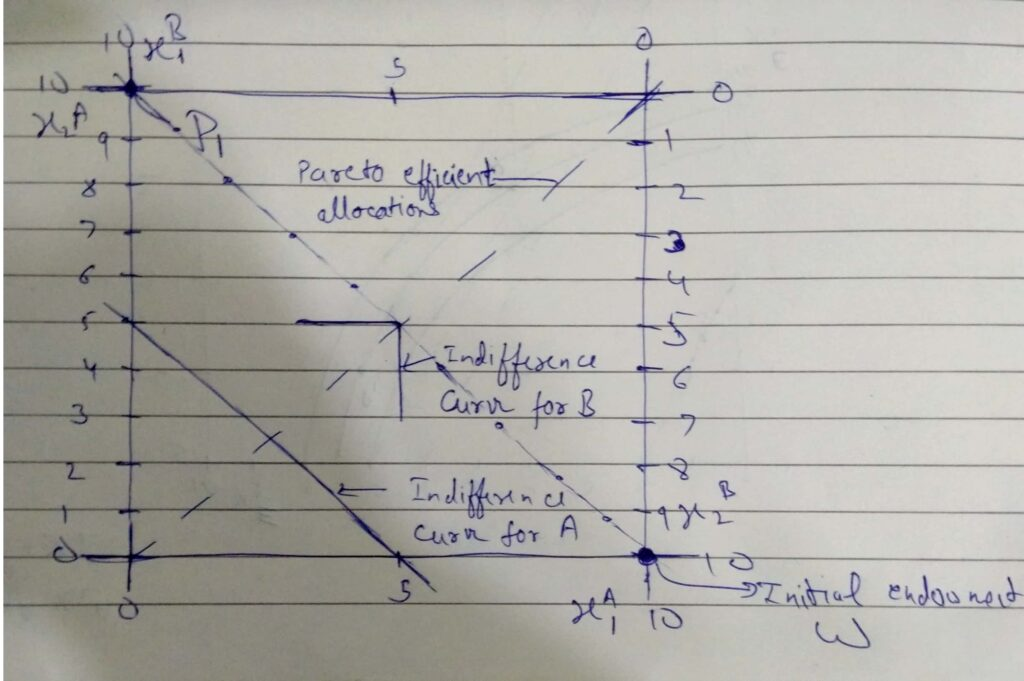## (c)

Yes, it is possible that in market equilibrium both the countries consume 5 million litres of each wine.

For country B both the goods are perfect substitutes thus the price ratios have to be 1. The quantities of both the goods are same in Country A and thus the prices of both of them will also have to be the same.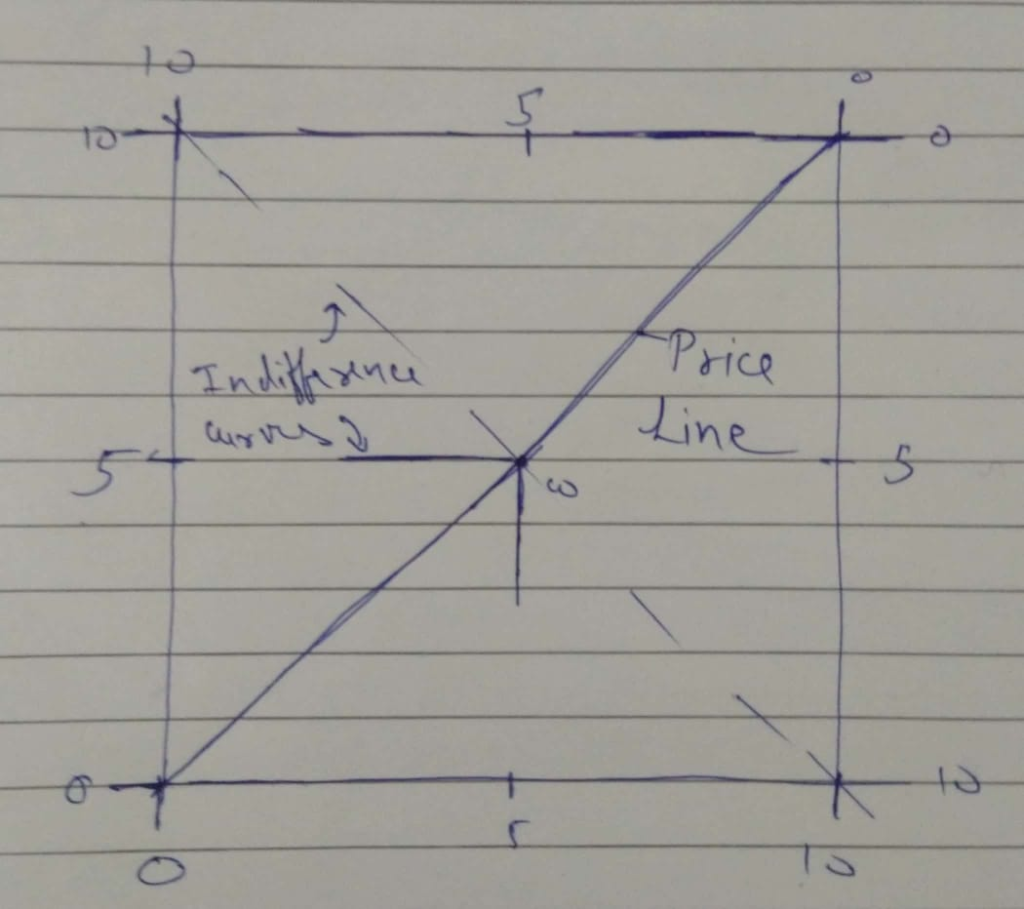## (d)

Yes, it is possible that in a market equilibrium, country A consumes 9 million litres of white wine and 9 million litres of red wine whereas country B consumes the remaining 1 million litres of white wine and 1 million litres of red wine.

For country B both the goods are perfect substitutes thus the price ratios have to be 1. The quantities of both the goods are same in Country A and thus the prices of both of them will also have to be the same.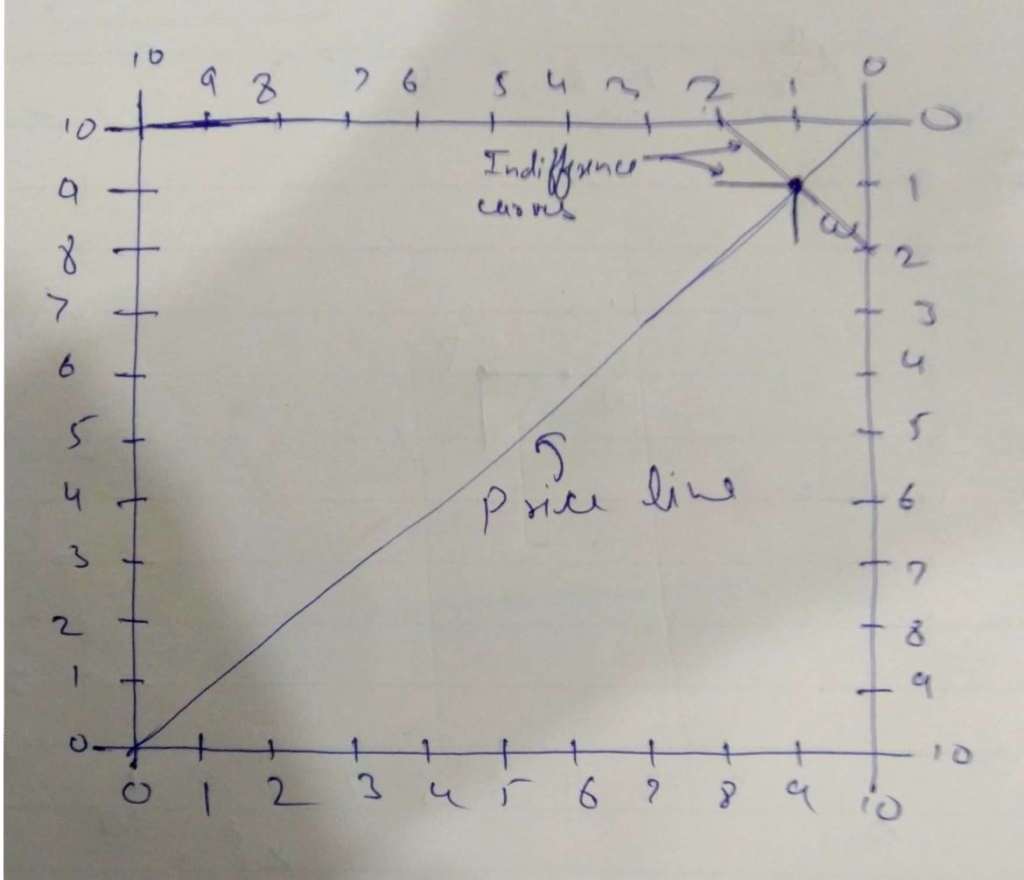Looking for best Economics Assignment Help. Whatsapp us at +16469488918 or chat with our chat representative showing on lower right corner or order from here. You can also take help from our Live Assignment helper for any exam or live assignment related assistance.